Next: Central schemes in two Up: Central schemes in one-space Previous: The second-order Nessyahu-Tadmor scheme

## The third-order central scheme

Following the framework outlined in §1.3.1, the upgrade to third-order central scheme consists of two main ingredients:

(i)
A third-order accurate, piecewise-quadratic polynomial reconstruction which enjoys desirable non-oscillatory properties;
(ii)
An appropriate quadrature rule to approximate the numerical fluxes along cells' interfaces.

Following , we proceed as follows. The piecewise-parabolic reconstruction takes the form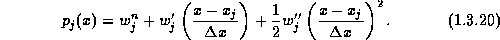Here,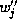are the (pointvalues of) the reconstructed second derivatives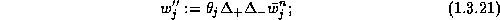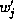are the (pointvalues of) the reconstructed slopes,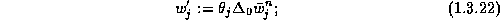and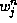are the reconstructed pointvalues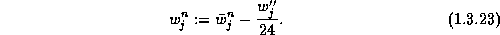Observe that, starting with third- (and higher-) order accurate methods, pointwise values cannot be interchanged with cell averages,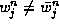.

Here,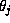are appropriate nonlinear limiters which guarantee the non-oscillatory behavior of the third-order reconstruction; its precise form can be found in , . They guarantee that the reconstruction (1.3.20) is non-oscillatory in the sense that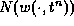-- the number of extrema of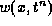, does not exceed that of its piecewise-constant projection,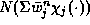,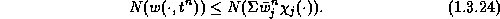Next we turn to the evolution of the piecewise-parabolic reconstructed solution. To this end we need to evaluate the staggered averages,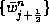, and to approximate the interface fluxes,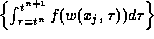.

With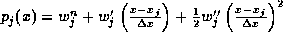specified in (1.3.20)-(1.3.23), one evaluates the staggered averages of the third order reconstruction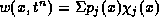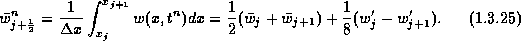Remarkably, we obtain here the same formula for the staggered averages as in the second-order cases, consult (1.3.17); the only difference is the use of the new limited slopes in (1.3.22),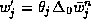.

Next, we approximate the (exact) numerical fluxes by Simpson's quadrature rule, which is (more than) sufficient for retaining the overall third-order accuracy,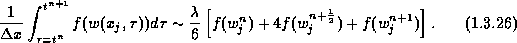This in turn, requires the three approximate pointvalues on the right,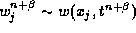for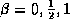. Following our approach in the second-order case, , we use Taylor expansion to predict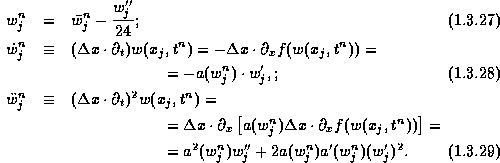In summary of the scalar setup, we end up with a two step scheme where, starting with the reconstructed pointvalues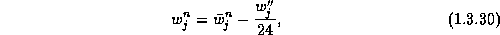we predict the pointvalues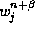by, e.g. Taylor expansions,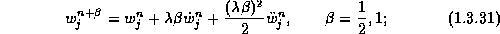this is followed by the corrector step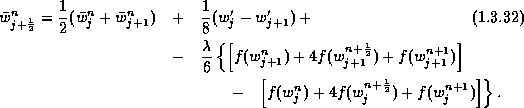In figure 1.3.2 we revisit the so called Woodward-Colella problem, , where we compare the second vs. the third-order results. The improvement in resolving the density field is evident.

We conclude this section with several remarks. Remarks.

1. Stability.

We briefly mention the stability results for the scalar central schemes. In the second order case, the NT scheme was shown to be both TVD and entropy stable in the sense of satisfying a cell entropy inequality - consult . The third-order scalar central scheme is stable in the sense of satisfying the NED property, (1.3.24), namely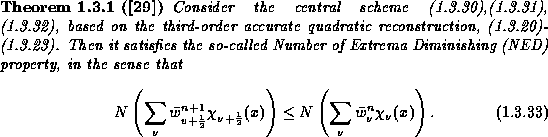2. Source terms, radial coordinates, ...

Extensions of the central framework which deal with both, stiff and non-stiff source terms can be found in ,, , . In particular, Kupferman in , developed the central framework within the radial coordinates which require to handle both - variable coefficients + source terms.

3. Higher order central schemes.

We refer to , where a high-order ENO reconstruction is realized by a staggered cell averaging. Here, intricate Riemann solvers are replaced by high order quadrature rules. and for this purpose, one can effectively use the RK method (rather than the Taylor expansion outlined above):

4. Taylor vs. Runge-Kutta.

The evaluations of Taylor expansions could be substituted by the more economical Runge-Kutta integrations; the simplicity becomes more pronounced with systems. A particular useful approach in this context was proposed in , using the natural continuous extensions of RK schemes.

5. Systems.

One of the main advantages of our central-staggered framework over that of the upwind schemes, is that expensive and time-consuming characteristic decompositions can be avoided. Specifically, all the non-oscillatory computations can be carried out with diagonal limiters, based on a
component-wise extension of the scalar limiters outlined above.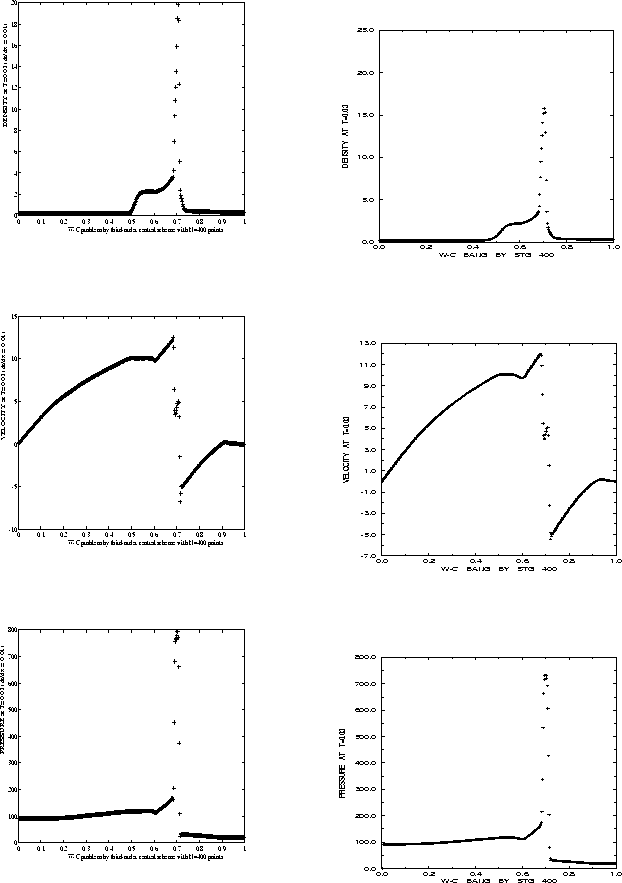Figure 1.3.5: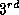vs.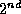order central schemes -- Woodward-Colella problem at t=0.03Next: Central schemes in two Up: Central schemes in one-space Previous: The second-order Nessyahu-Tadmor scheme